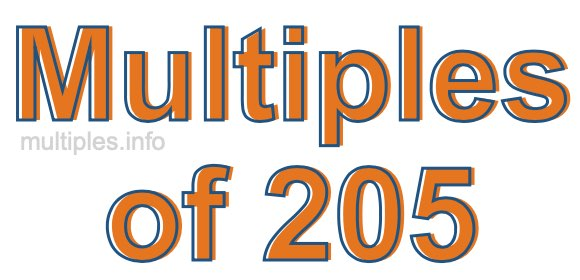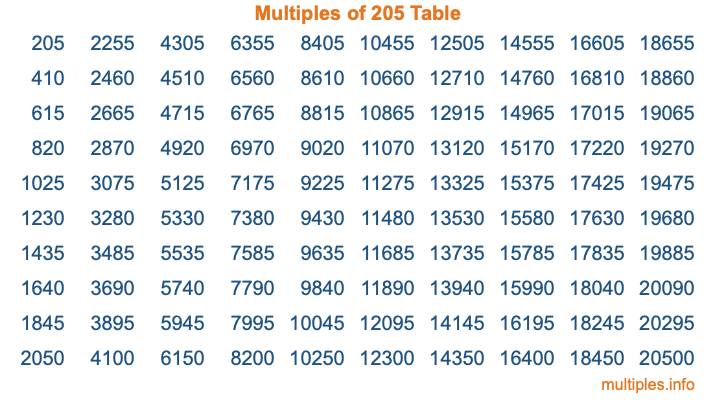Multiples of 205Welcome to the Multiples of 205 page. Here we will first teach you everything you will ever need to know about the multiples of 205, and then give you a study guide summary of everything we taught you to make sure you remember it all. Use this page to look up facts and learn information about the multiples of 205. This page will make you a multiples of two hundred five expert!

Definition of Multiples of 205
Multiples of 205 are all the numbers that when divided by 205 equal an integer. Each of the multiples of 205 are called a multiple. A multiple of 205 is created by multiplying 205 by an integer.

Therefore, to create a list of multiples of 205, you start with 1 multiplied by 205, then 2 multiplied by 205, then 3 multiplied by 205, and so on for as long as you want. Thus, the list of the first five multiples of 205 is 205, 410, 615, 820, and 1025. To see a larger list of multiples of 205, see the printable image of Multiples of 205 further down on this page. We also have a category where you can choose any nth multiple of 205.

Multiples of 205 Checker
The Multiples of 205 Checker below checks to see if any number of your choice is a multiple of 205. In other words, it checks to see if there is any number (integer) that when multiplied by 205 will equal your number. To do that, we divide your number by 205. If the the quotient is an integer, then your number is a multiple of 205.

Is  a multiple of 205?

Least Common Multiple of 205 and ...
A Least Common Multiple (LCM) is the lowest multiple that two or more numbers have in common. This is also called the smallest common multiple or lowest common multiple and is useful to know when you are adding our subtracting fractions. Enter one or more numbers below (205 is already entered) to find the LCM.

Check out our LCM Calculator if you need more details about the Least Common Multiple or if you need the LCM for different numbers for adding and subtraction fractions.

nth Multiple of 205
As we stated above, 205 is the first multiple of 205, 410 is the second multiple of 205, 615 is the third multiple of 205, and so on. Enter a number below to find the nth multiple of 205.

th multiple of 205

Multiples of 205 vs Factors of 205
205 is a multiple of 205 and a factor of 205, but that is where the similarities end. All postive multiples of 205 are 205 or greater than 205. All positive factors of 205 are 205 or less than 205.

Below is the beginning list of multiples of 205 and the factors of 205 so you can compare:

Multiples of 205: 205, 410, 615, 820, 1025, etc.

Factors of 205: 1, 5, 41, 205

As you can see, the multiples of 205 are all the numbers that you can divide by 205 to get a whole number. The factors of 205, on the other hand, are all the whole numbers that you can multiply by another whole number to get 205.

It's also interesting to note that if a number (x) is a factor of 205, then 205 will also be a multiple of that number (x).

Multiples of 205 vs Divisors of 205
The divisors of 205 are all the integers that 205 can be divided by evenly. Below is a list of the divisors of 205.

Divisors of 205: 1, 5, 41, 205

The interesting thing to note here is that if you take any multiple of 205 and divide it by a divisor of 205, you will see that the quotient is an integer.

Multiples of 205 Table
Below is an image of the first 100 multiples of 205 in a table. The table is in chronological order, column by column. The first column has the first ten multiples of 205, the second column has the next ten multiples of 205, and so on.The Multiples of 205 Table is also referred to as the 205 Times Table or Times Table of 205. You are welcome to print out our table for your studies.

Negative Multiples of 205
Although not often discussed or needed in math, it is worth mentioning that you can make a list of negative multiples of 205 by multiplying 205 by -1, then by -2, then by -3, and so on, to get the following list of negative multiples of 205:

-205, -410, -615, -820, -1025, etc.

Multiples of 205 Summary
Below is a summary of important Multiples of 205 facts that we have discussed on this page. To retain the knowledge on this page, we recommend that you read through the summary and explain to yourself or a study partner why they hold true.

There are an infinite number of multiples of 205.

A multiple of 205 divided by 205 will equal a whole number.

205 divided by a factor of 205 equals a divisor of 205.

The nth multiple of 205 is n times 205.

The largest factor of 205 is equal to the first positive multiple of 205.

205 is a multiple of every factor of 205.

205 is a multiple of 205.

A multiple of 205 divided by a divisor of 205 equals an integer.

205 divided by a divisor of 205 equals a factor of 205.

Any integer times 205 will equal a multiple of 205.

Multiples of a Number
Here you can get the multiples of another number, all with the same attention to detail as we did for multiples of 205 on this page.

Multiples of
Multiples of 206
Did you find our page about multiples of two hundred five educational? Do you want more knowledge? Check out the multiples of the next number on our list!

Copyright  |   Privacy Policy  |   Disclaimer  |   Contact